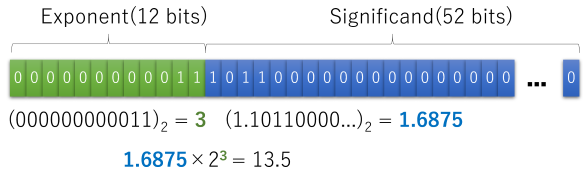# Floating-Point Numbers

Time Limit : 8 sec, Memory Limit : 262144 KB
Japanese version is here

### Floating-Point Numbers

In this problem, we consider floating-point number formats, data representation formats to approximate real numbers on computers.

Scientific notation is a method to express a number, frequently used for numbers too large or too small to be written tersely in usual decimal form. In scientific notation, all numbers are written in the form m × 10e. Here, m (called significand) is a number greater than or equal to 1 and less than 10, and e (called exponent) is an integer. For example, a number 13.5 is equal to 1.35 × 101, so we can express it in scientific notation with significand 1.35 and exponent 1.

As binary number representation is convenient on computers, let's consider binary scientific notation with base two, instead of ten. In binary scientific notation, all numbers are written in the form m × 2e. Since the base is two, m is limited to be less than 2. For example, 13.5 is equal to 1.6875 × 23, so we can express it in binary scientific notation with significand 1.6875 and exponent 3. The significand 1.6875 is equal to 1 + 1/2 + 1/8 + 1/16, which is 1.10112 in binary notation. Similarly, the exponent 3 can be expressed as 112 in binary notation.

A floating-point number expresses a number in binary scientific notation in finite number of bits. Although the accuracy of the significand and the range of the exponent are limited by the number of bits, we can express numbers in a wide range with reasonably high accuracy.

In this problem, we consider a 64-bit floating-point number format, simplified from one actually used widely, in which only those numbers greater than or equal to 1 can be expressed. Here, the first 12 bits are used for the exponent and the remaining 52 bits for the significand. Let's denote the 64 bits of a floating-point number by b64...b1. With e an unsigned binary integer (b64...b53)2, and with m a binary fraction represented by the remaining 52 bits plus one (1.b52...b1)2, the floating-point number represents the number m × 2e.

We show below the bit string of the representation of 13.5 in the format described above.In floating-point addition operations, the results have to be approximated by numbers representable in floating-point format. Here, we assume that the approximation is by truncation. When the sum of two floating-point numbers a and b is expressed in binary scientific notation as a + b = m × 2e (1 ≤ m < 2, 0 ≤ e < 212), the result of addition operation on them will be a floating-point number with its first 12 bits representing e as an unsigned integer and the remaining 52 bits representing the first 52 bits of the binary fraction of m.

A disadvantage of this approximation method is that the approximation error accumulates easily. To verify this, let's make an experiment of adding a floating-point number many times, as in the pseudocode shown below. Here, s and a are floating-point numbers, and the results of individual addition are approximated as described above.

```s := a
for n times {
s := s + a
}
```

For a given floating-point number a and a number of repetitions n, compute the bits of the floating-point number s when the above pseudocode finishes.

### Input

The input consists of at most 1000 datasets, each in the following format.

n
b52...b1

n is the number of repetitions. (1 ≤ n ≤ 1018) For each i, bi is either 0 or 1. As for the floating-point number a in the pseudocode, the exponent is 0 and the significand is b52...b1.

The end of the input is indicated by a line containing a zero.

### Output

For each dataset, the 64 bits of the floating-point number s after finishing the pseudocode should be output as a sequence of 64 digits, each being 0 or 1 in one line.

### Sample Input

```1
0000000000000000000000000000000000000000000000000000
2
0000000000000000000000000000000000000000000000000000
3
0000000000000000000000000000000000000000000000000000
4
0000000000000000000000000000000000000000000000000000
7
1101000000000000000000000000000000000000000000000000
100
1100011010100001100111100101000111001001111100101011
123456789
1010101010101010101010101010101010101010101010101010
1000000000000000000
1111111111111111111111111111111111111111111111111111
0
```

### Output for the Sample Input

```0000000000010000000000000000000000000000000000000000000000000000
0000000000011000000000000000000000000000000000000000000000000000
0000000000100000000000000000000000000000000000000000000000000000
0000000000100100000000000000000000000000000000000000000000000000
0000000000111101000000000000000000000000000000000000000000000000
0000000001110110011010111011100001101110110010001001010101111111
0000000110111000100001110101011001000111100001010011110101011000
0000001101010000000000000000000000000000000000000000000000000000
```

Source: ACM International Collegiate Programming Contest , Japan Domestic Contest, Yokohama, Japan, 2018-07-6
https://icpc.iisf.or.jp/2018-yokohama/